# Homological algebra

(diff) ← Older revision | Latest revision (diff) | Newer revision → (diff)

The branch of algebra whose main study is derived functors on various categories of algebraic objects (modules over a given ring, sheaves, etc.).

One of the origins of homological algebra is the (singular) homology theory of topological spaces. In this, to each topological spaceis associated a sequence of Abelian groups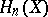(homology groups), while to a continuous mappingof spaces is associated a set of homomorphisms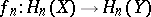of the homology groups. Each-dimensional singular simplexof a topological spacehas a boundary consisting of singular simplices of dimension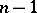. Ifis the free Abelian group generated by all the-dimensional simplices, then the functionwhich assigns to eachthe alternating sumof its boundary simplices defines a homomorphism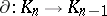so thatis a complex of Abelian groups, and a continuous mapping of spaces induces homomorphisms of their respective complexes. Certain properties of the spaceor the mappingsmay be deduced from the properties of the homology groups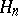of this complex or from the corresponding homomorphisms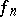of these homology groups. This makes it possible, in a number of cases, to reduce the study of topological objects to the study of certain algebraic objects, as is done in analytic geometry (with the difference that the transition from geometry to algebra in homology theory is irreversible).

In algebra, in turn, in the theory of groups (cf. Extension of a group), one studies in fact the first and the second homology and cohomology groups. Extensive preparatory material was developed in the theory of associative algebras, the theory of Lie algebras, the theory of finite-dimensional algebras, the theory of rings, and the theory of quadratic forms.

The language of homological algebra arose mainly from the process of studying homology groups. There appeared arrows as symbols for mappings and commutative diagrams (if in a diagram any two paths with common beginning and end give rise to the same composite mapping, then the diagram is said to be commutative). Sequences of homomorphisms in which the kernel of each outgoing homomorphism coincides with the image of the incoming one were encountered; such sequences are called exact (cf. Exact sequence). It became customary to specify mathematical objects together with their mappings; the correspondences most preferred were those between objects that preserve the mappings. These correspondences became known as functors (cf. Functor). The principal advantages of this language — the amount of information conveyed, naturalness and clarity — were soon recognized. For example, the language of homological algebra was employed  in the axiomatic exposure of the fundamentals of algebraic topology. Nowadays this language is used in numerous studies, including those not employing homological methods.

By the mid-1940s homological algebra had become an independent branch of algebra. The principal domain of application of homological algebra is the category of modules over a ring. Most of the results known for modules may be applied to Abelian categories with certain restrictions (this is because such categories are imbeddable into categories of modules). In the most fruitful extension of the domain of application of homological algebra , the latter was extended so as to apply to arbitrary Abelian categories with enough injective objects, and became applicable to arithmetical algebraic geometry and to the theory of functions in several variables (cf. Grothendieck category).

The principal functors of homological algebra are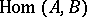(the group of homomorphisms of a moduleinto a module) and the tensor product of modules,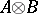. The base of the theory is the study of derived functors (cf. Derived functor), which may be constructed as follows. An arbitrary modulecan be represented as a quotient module of a free module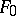, after which a similar representation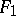is considered for the kernel of the preceding representation, etc. One thus obtains an exact sequence: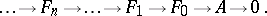An exact sequencewhere all the modulesare projective is called a projective resolution of the module. The application of a covariant additive functoryields a complex, whose homology groups are known as the left derived functors of; they are denoted by. A dual construction (for a contravariant functor) — or by using injective modules and injective resolutions (for a covariant functor) — is performed to obtain the right derived functors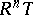. In a certain sense, the derived functors are a measure of the deviation of the functor from exactness. They are not affected by the arbitrariness involved in the construction of a resolution. To each exact sequence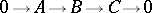correspond two infinite exact sequences of derived functors: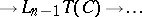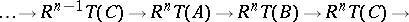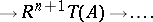The following notation is accepted for the derived functors of basic functors: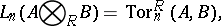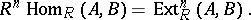Both these functors are functors of two argumentsand, and for this reason the construction of the derived functor as described above is not directly applicable to them. In such a case one can fix one argument and construct a resolution for the other or, having constructed resolutions of both arguments, one can construct a binary complex. The same result will be obtained in all cases. The groupis isomorphic to the group of extensions of the moduleby the module(and in this form it has been studied for a long time). The establishment of the new relations considerably extended and advanced the theory of extensions of modules. The group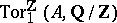is the torsion part of a group. The generalization of this observation resulted in the development of the general theory of torsion.

The homology theory of algebraic systems forms part of the general scheme of derived functors. Thus, let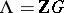be the group ring (cf. Group algebra) of a multiplicative groupover the ring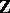of integers, and letbe a left and letbe a right-module. The study of the groups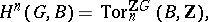whereis considered as a trivial left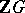-module, is the theory of homology and cohomology of groups. Letbe a Lie algebra over a field, let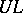be its universal enveloping algebra, and letbe a-module. The study of the groups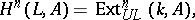whereis considered as a trivial-module, is the theory of cohomology of Lie algebras. Appropriate cohomology and homology groups of monoids, Abelian groups, algebras, graded algebras, rings, etc., are defined in a similar manner. The guideline in each case is the fact that the second cohomology group is the group of extensions for the type of algebraic systems under consideration.

In turn, the homology groups of algebraic systems form the subject of study of relative homological algebra.

In concrete cases derived functors of functors are usually computed by means of an explicit resolution. The resolution may be finite (e.g. the length of the resolution of an arbitrary Abelian group does not exceed one). There has long been interest in the length of the shortest resolution (this length is called the homological dimension). The first significant result in this direction is the Hilbert theorem on syzygies (end of the 19th century). Homological dimension theory is one of the actively-developing branches of homological algebra. The transition from modules with various restrictions of finiteness to the general case is often performed with the aid of the inductive limit and projective limit functors,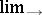and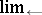. Thus, any group is the inductive limit of its finitely-generated subgroups. Every compact totally-disconnected group is representable as the projective limit of its finite quotient groups. Interest in these groups stems from their connection with Galois theory. The derived functors of these functors are used in homological dimension theory.

Derived functors for non-additive functors have been studied (e.g. functors which relate an Abelian group to its group ring or to its symmetric algebra).

The principal means of computations in homological algebra, other than the resolutions already mentioned, are spectral sequences (cf. Spectral sequence) and the homology product. The former, which are a most powerful tool in the study of derived functors, approximate the homology groups of a group by the homology groups of a subgroup and a quotient group of it. The homology product relates to the study of homomorphisms of the type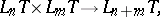which combine derived functors with one another.

Methods of homological algebra are now extensively employed in very different branches of mathematics, like functional analysis, the theory of functions of a complex variable, differential equations, etc. Branches of algebra such as algebraic-theory, algebraic geometry or algebraic number theory would be unthinkable without homological algebra.

How to Cite This Entry:
Homological algebra. Encyclopedia of Mathematics. URL: http://encyclopediaofmath.org/index.php?title=Homological_algebra&oldid=12172
This article was adapted from an original article by V.E. GovorovA.V. Mikhalev (originator), which appeared in Encyclopedia of Mathematics - ISBN 1402006098. See original article